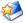# [原创]研制10吨级的重型通讯卫星与导航卫星已经是历史的必然发展趋势

[size=10.5]研制[size=10.5]10[size=10.5]吨级的重型通讯卫星与导航卫星已经是历史的必然发展趋势[size=10.5]
[size=10.5]
[size=10.5]鉴于目前捕食者之类中远程查打一体化无人攻击机在中东与非洲辽阔地区的巨大反恐打击作用，与及全球鹰无人侦察机在美国维持实时“全球态势感知”方面的中枢地位。重型移动通讯卫星与重型宽带通讯卫星的政府需求在未来四、五十年内都是始终存在的。那怕太空探索公司的低轨道“星链通讯卫星”发展起来，[size=10.5]GEO[size=10.5]轨道重型通讯卫星仅仅作为政府与军用上的备份，都是必须存在的。[size=10.5]
[size=10.5]
[size=10.5]第一，政府与军用部门外包卫星通信服务已经成为全球发展趋势。[size=10.5]
[size=10.5]
[size=10.5]事实上军用战术、战略无人机对移动通讯卫星与宽带通讯卫星的需求远远还没有发展到尽头之时。那怕即使是对美国政府而言，在[size=10.5]AEHF[size=10.5]、[size=10.5]MOUS[size=10.5]与[size=10.5]WGS[size=10.5]三大军事通讯卫星系统建设完成之后，未来十年后的政府与军用移动通讯与宽带通讯采取什么的途径来解决，现在相关各方还在探索讨论之中。如果决心通过采购商业通讯卫星的方案来解决，这也将是新增[size=10.5]10[size=10.5]至[size=10.5]15[size=10.5]颗重型通讯卫星的巨大市场容量。[size=10.5]
[size=10.5]
[size=10.5]早期洛马公司在[size=10.5]TSAT[size=10.5]军用通讯卫星项目中止之后，就建议美国政府采购洛马公司的“改进型[size=10.5]AEHF[size=10.5]军用通讯卫星（重达[size=10.5]11[size=10.5]吨以上、计划要使用德尔塔[size=10.5]4H[size=10.5]重型火箭来发射）”来填补[size=10.5]TSAT[size=10.5]军用通讯卫星项目中止后的政府需求，但目前己经不了了之。[size=10.5]
[size=10.5]
[size=10.5]鉴于未来全球政府与军用部门对移动通讯卫星与宽带通讯卫星所存在的明确无误的庞大市场需求，但目前各国政府都有计划向商业通讯卫星市场采购越来越多的宽带服务（即政府与军方不再独立运营庞大的通讯卫星系统，而是将政府服务向商业通讯卫星公司进行外包）。因此象欧洲卫星组织、[size=10.5]SES[size=10.5]、海事卫星组织、国际卫星组织、美洲卫星组织、美国卫讯与中国卫通这样的全球主流商业通讯卫星运营商的市场机会都明确无误地存在的。高凉陈君估算下，那怕单纯维持目前的服务规模。仅仅是美国、欧洲与中国这三大集团的政府与军用移动通讯卫星、宽带通讯卫星服务改为向商业市场进行采购，未来十五年内就存在至少[size=10.5]50[size=10.5]颗的重型通讯卫星市场增量需求。[size=10.5]
[size=10.5]
[size=10.5]而纯民用市场再什么萎缩，未来十五年内全球也至少存在[size=10.5]150[size=10.5]颗（即每年那怕维持仅仅[size=10.5]10[size=10.5]颗的水平）重型通讯卫星的市场需求。两者合计全球重型通讯卫星未来[size=10.5]15[size=10.5]年内的市场需求就至少为[size=10.5]200[size=10.5]颗了。因此，近期国际市场上民用通讯卫星需求的暂时性市场萎缩说明不了什么。由于政府与军事部门对移动通讯卫星与宽带通讯卫星的需求是硬性存在的（典型的刚需），而且末来几十时间里象空客防务、中国航天科技公司、洛马、波音与三菱电机的通讯卫星生产部门也绝对不可能停产关闭。因此各大国政府那怕仅仅为了养活自己的通讯卫星制造公司，也必须让政府或者本国的卫星运营商定期向本国的卫星生产商采购一批通讯卫星产品。有政府机构在后面进行背书支持，卫星生产商那怕生产出的卫星是重达[size=10.5]10[size=10.5]吨级的重型通讯卫星，也是一样不必担心卖不出去。[size=10.5]
[size=10.5]
[size=10.5]当然“政府采购版”的重型通讯卫星，价格也不能够太贵，高大上的技术不能够堆砌太多。高凉陈君认为全球各大卫星生产商大力生产[size=10.5]9[size=10.5]至[size=10.5]10[size=10.5]吨级别的全化学推进或者混电推进重型通讯卫星都是完全可行的未来发展方向。[size=10.5]

[size=10.5]第二，全球导航卫星增重的发展态势也越来越明显。[size=10.5]
[size=10.5]
[size=10.5]同样，导航卫星全球增重的发展态势也越来越明显。美国的[size=10.5]GPS[size=10.5]卫星一代就比一代重。[size=10.5]GPS3[size=10.5]代导航卫星的重量就有[size=10.5]4[size=10.5]吨以上。因此，高凉陈君认为中国的下一代北斗全球导航卫星与欧洲的下一代伽利略导航卫星都“大概率”会走美国目前[size=10.5]GPS3[size=10.5]代卫星增重的发展路径，未来也一样会增重到[size=10.5]4[size=10.5]、[size=10.5]5[size=10.5]吨的级别水平。因为导航卫星本质上也是通讯卫星的一种，导航卫星的发射功率越大，地面上的导航卫星接收终端的体积与功率就可以做得越小。因此美国的[size=10.5]GPS[size=10.5]导航卫星越做越重是有其事情根据的。而且洛马公司生产的[size=10.5]GPS3[size=10.5]导航卫星就是基于[size=10.5]A2100[size=10.5]公用卫星平台来研制的，市场采购价格也要[size=10.5]4[size=10.5]、[size=10.5]5[size=10.5]亿美元一颗。[size=10.5]
[size=10.5]
[size=10.5]与之相反，目前欧洲的伽利略导航卫星，中国的北斗全球组网导航卫星与俄罗斯的格洛纳斯导航卫星的重量还停留于只有[size=10.5]1[size=10.5]吨左右的出头，还没有象美国那样越做越重。核心原因就是欧、中、俄三大集团现在只能够去尽快解决自己独立自主导航卫星系统的“有无问题”，暂时还无法象美国人那样顾及得上“好坏”。因此也只能去生产重量只有[size=10.5]1[size=10.5]吨左右的小型导航卫星来勉强应付一下就了事。毕竟如果欧、中、俄一上马全球组网版导航卫星就是目前美国[size=10.5]GPS3[size=10.5]代那样重达[size=10.5]4[size=10.5]吨以上的“高大上”产品，仅仅是昂贵的运载火箭发射成本也不是欧、中、俄三集团现在就轻易能够承受得起的。[size=10.5]
[size=10.5]
[size=10.5]问题是随着欧、中、俄初始版（至少[size=10.5]24[size=10.5]颗）全球导航卫星组网建设的完成，未来下一代的导航卫星由于可以分期、分阶段（以[size=10.5]8[size=10.5]颗为一期）来研制发射，投资压力不象初始版那么大。那么欧、中、俄的补网导航卫星也一样会象美国的[size=10.5]GPS3[size=10.5]代那样，也会逐渐走重量越做越大的“高大上”发展路径。高凉陈君就认为，除了目前经济上贫困的俄罗斯外，中国与欧洲的下一代全球组网导航卫星的重量也必然会与美国的[size=10.5]GPS3[size=10.5]代导航卫星一样，重量也至少是[size=10.5]4[size=10.5]吨以上的“高大上”的产品。按导航卫星全球组网一般要发射[size=10.5]24[size=10.5]颗卫星的市场需求来分析。如果中国与欧洲的下一代全球组网导航卫星也是采用至少[size=10.5]4[size=10.5]吨级的方案的话，那么即使按照一箭双星的方式来发射，也需要使用[size=10.5]GTO[size=10.5]运力[size=10.5]9[size=10.5]至[size=10.5]10[size=10.5]吨级的阿里安[size=10.5]6[size=10.5]（[size=10.5]4[size=10.5]助推器版本）与缩小版长征五号火箭各[size=10.5]12[size=10.5]枚。[size=10.5]
[size=10.5]
[size=10.5]事实上美国在发射[size=10.5]GPS[size=10.5]导航卫星时，就采购过宇宙神[size=10.5]551[size=10.5]火箭以一箭双星的方式来执行过发射任务。[size=10.5]
[size=10.5]
[size=10.5]同样，随着全球导航卫星发射功率与功能的进一步增强，末来[size=10.5]GPS4[size=10.5]代、[size=10.5]GPS5[size=10.5]代导航卫星的重量做到[size=10.5]9[size=10.5]至[size=10.5]10[size=10.5]吨级都是顺理成章的事情。一旦如此，中国、俄罗斯与欧洲们为了紧跟美国人的前进步伐，未来也一样必须研制重达[size=10.5]9[size=10.5]至[size=10.5]10[size=10.5]吨级的重型导航卫星。[size=10.5]

打赏
收藏文本
0
0
2019/2/7 13:08:18

## 网友回复

左箭头-小图标

[size=10.5]第三，人们绝对不能够先入为主地认为导航卫星只是重量只有[size=10.5]1[size=10.5]吨多的“小不点”。[size=10.5]
[size=10.5]
[size=10.5]美国历年来[size=10.5]GPS[size=10.5]导航卫星越做越重、越做越大的发展态势表明，重量只有[size=10.5]1[size=10.5]吨左右的导航卫星只是“穷”国家的玩具而已。土豪国家的先进导航卫星重量没有[size=10.5]4[size=10.5]、[size=10.5]5[size=10.5]吨都不好意思出门来见人。而在地球这个行星上，研制与应用导航卫星的经验谁也比不过美国。因此高凉陈君认为美国人持续做大[size=10.5]GPS[size=10.5]导航卫星就必然有他的深层理由，只是具体原因还不为外界所知晓与理解而已。[size=10.5]
[size=10.5]
[size=10.5]高凉陈君当然不会不知道长三乙[size=10.5]/[size=10.5]远征上面级火箭所发射的北斗导航卫星是直接入轨的事实。问题是即使增加上远征上面级所取代的卫星自身升轨功能模块，中国北斗全球组网导航卫星的重量也只有[size=10.5]2[size=10.5]点[size=10.5]5[size=10.5]吨左右而已，重量比美国的[size=10.5]GPS3[size=10.5]代导航卫星的[size=10.5]4[size=10.5]吨级还是要轻得多。[size=10.5]
[size=10.5]
[size=10.5]第四，高凉陈君现在对中国航天事业最重要的贡献有两大方面。[size=10.5]
[size=10.5]
[size=10.5]一，就是主张中国必须要研制[size=10.5]LEO[size=10.5]运力[size=10.5]35[size=10.5]至[size=10.5]80[size=10.5]吨区间、[size=10.5]GTO[size=10.5]运力[size=10.5]20[size=10.5]至[size=10.5]40[size=10.5]吨区间的“中间运力型火箭”。并详细分析与构建中国“中间运力型火箭”未来的长久生存与使用运营模型。[size=10.5]
[size=10.5]
[size=10.5]高凉陈君的这一中间运力型火箭研制构想现在就已经转化为[size=10.5]921[size=10.5]办公室提出的‘[size=10.5]5[size=10.5]米直径煤油主芯级新载人火箭研制方案”了。懂得人自然就懂，多说无益。[size=10.5]
[size=10.5]
[size=10.5]二，就是主张中国必须要研制[size=10.5]GTO[size=10.5]运力[size=10.5]9[size=10.5]至[size=10.5]10[size=10.5]吨区间的“缩小版长征五号火箭”。现在高凉陈君也在详细分析此一款火箭未来长久的生存与使用运营模型。[size=10.5]
[size=10.5]
[size=10.5]高凉陈君现在认为未来中国研制下一代北斗全球组网导航卫星时，“大概率”就会重走美国人今天研制[size=10.5]4[size=10.5]吨级的[size=10.5]GPS3[size=10.5]代大型导航卫星的技术发展路径。而重量达[size=10.5]4[size=10.5]吨级的下一代北斗全球组网导航卫星就非常适合[size=10.5]GTO[size=10.5]运力只有[size=10.5]9[size=10.5]至[size=10.5]10[size=10.5]吨的“缩小版长征五号火箭”以一箭双星的方式来执行发射任务。[size=10.5]
[size=10.5]
[size=10.5]因此，至少对于中国的航天事业而言，高凉陈君就率先提出了“中间运力型火箭”的发展概念与使用运营模型与“缩小版长征五号火箭”的发展概念与使用运营模型这两大著名观点。而为了中国“缩小版长征五号火箭”未来的长久生存与使用运营，高凉陈君现在就在详细论述未来中国研制重量高达[size=10.5]9[size=10.5]至[size=10.5]10[size=10.5]吨级的基于东方红五号公用平台的重型通讯卫星与重量高达[size=10.5]4[size=10.5]至[size=10.5]5[size=10.5]吨的下一代北斗全球组网导航卫星的“必要性、合理性与可行性”。其背后的深层思考构建方式，其实与早年高凉陈君为了“长久养活”中国的中间运力型火箭，进而主张中国必须“基于月球轨道对接方案”来实施执行中国未来的载人登月工程是完全一模一样的。[size=10.5]
[size=10.5]
[size=10.5]第五，未来全球民用商业通讯卫星市场越是萎缩，全球导航卫星的重量就越有必要与动力去“全力做大”。力求实现“东边不亮西边、外墙损失内墙补”的目标。[size=10.5]
[size=10.5]
[size=10.5]否则未来空客防务、洛马、波音与中国航天科技公司的卫星制造部门“吃什么”？！难道要通通都去“吃西北风”？！[size=10.5]
[size=10.5]
[size=10.5]因此，如果高凉陈君是洛马、波音与空客防务的老总，我现在也恨不得要将导航卫星的重量通通做大到[size=10.5]10[size=10.5]吨级的巨大规模。力求要做到“十年不发市、发市就要足够吃十年”的程度。毕竟全球组网的导航卫星全部轮换一遍就是高达[size=10.5]24[size=10.5]颗的“大订单”，不乘这好不容易才逮住一个大单的机会“狠狠地吃个够、吃个饱”，这简直就是在犯罪。[size=10.5]
[size=10.5]
[size=10.5]导航卫星的生产商“全力做大”导航卫星，对于卫星生产商、运载火箭生产商与广大的民间普通使用者而言都是典型的“你好、我好、大家好”的共赢事情。否则导航卫星做得太小，卫星发射功率太弱。用户搜星服务时总是提示“[size=10.5]GPS[size=10.5]无信号、[size=10.5]GPS[size=10.5]信号弱”，这才是真正的大悲剧。因此，高凉陈君认为未来各国的“中间运力型火箭”大规模投入使用之后，全球研制重量达[size=10.5]10[size=10.5]吨级的重型导航卫星就将会成为一种必然的决策（可以通过[size=10.5]GTO[size=10.5]运力达[size=10.5]24[size=10.5]吨的中间运力型火箭以一箭双星的方式来执行发射任务）。而且作为超级大国向全球社会提供的一种极其重要的“政府级免费太空共公基础设施工程（另外一种是气象卫星）”，相关各方都有持续做大导航卫星的“冲动”。理由就有下面[size=10.5]3[size=10.5]点。[size=10.5]
[size=10.5]
[size=10.5]1[size=10.5]，对于政府机构而言这样做能够收获广泛的社会赞赏。至少钱都是用于做了真真正正的“实事（钱不是用去填老鼠洞了、政绩天下人人可见）”。[size=10.5]
[size=10.5]
[size=10.5]2[size=10.5]，对于卫星生产商与运载火箭生产商而言，则收获了真真正正的经济利益。[size=10.5]
[size=10.5]
[size=10.5]3[size=10.5]，对于广大社会民众而言，则实实在在地收获了高质量的政府免费太空公共气象预报与导航定位服务。[size=10.5]
[size=10.5]
[size=10.5]目前美国最重的[size=10.5]GEO[size=10.5]轨道气象卫星已经要使用到宇宙神[size=10.5]551[size=10.5]火箭来发射了。因此未来全球气象卫星与导航卫星的重量做到[size=10.5]10[size=10.5]吨级都是完全可以设想的事情。因为相关各方都有真正的“冲动与企图”去支持这样做（也能够实现多方目标的真正共赢）。成果也能够为“方方面面”的势力所乐观其成。[size=10.5]
[size=10.5]
[size=10.5]陈天（高凉陈君）[size=10.5]
[size=10.5]
[size=10.5]2019-2-7

2019/2/7 13:11:28

总页数11页 [共有2条记录] 分页：

1
对[原创]研制10吨级的重型通讯卫星与导航卫星已经是历史的必然发展趋势回复